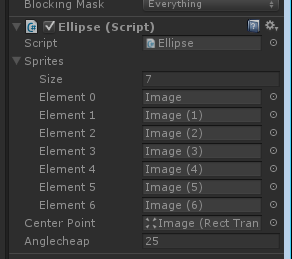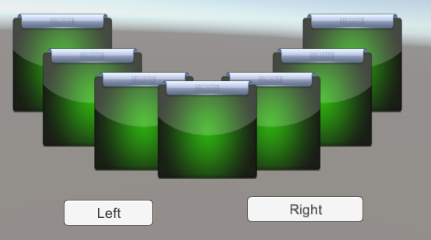# unity3d5.1物体椭圆旋转选择界面实现（一）

  偶然在群里看到有人拿了一张椭圆物体旋转选择界面来问怎么实现（也不知道这样描述对不对，反正是椭圆的，而且还是旋转的，类似于关卡和角色选择），于是

想了下自己写了一个类似的功能，方法可能不是很完美，但是也算是一个思路吧，就当练练手熟悉unity。原图如下：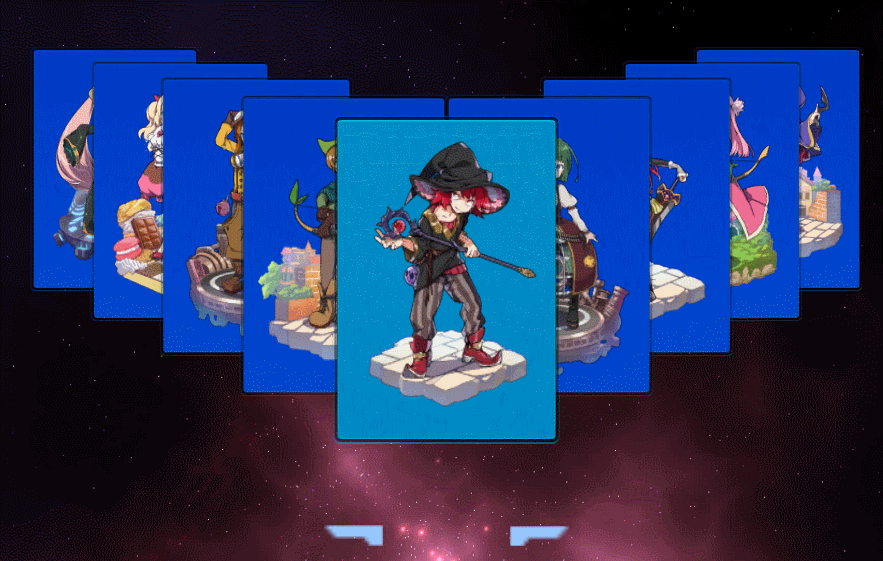附自己完成后的效果图：

遗憾的是不会做动态图片，也不知道大神写博客是怎么弄运行代码时的动态效果图，只能附上渣渣图片了~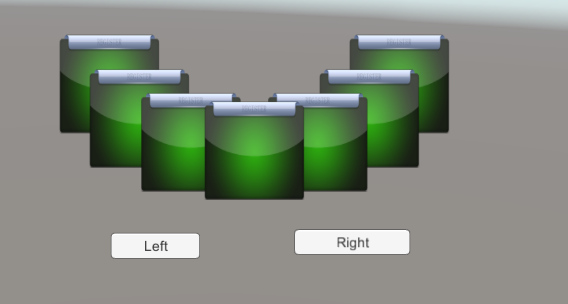基本效果能够达到一致，但是只是直接移动，原本是想实现绕椭圆轨迹移动，这里不得不说，unity动作机制几乎没有，原本api就难找，自己找半天连一个move的函

数都没有，如果不去找一个插件，只能通过update每一帧去手动设置GameObject的位置来达到move的效果，原本我以为是能够像cocos那样，最起码，moveto，得有，

### 一设计思路

• 方块的摆布：

首先需要明确的是方块的排布问题，摆布有多种方式，可以先将所有的方块保存到容器，之后按照一定的坐标差值来递增，递减这些方块的摆放位置，最后达到

该视觉效果，只是在排列的过程中需要长时间的调试效果，因而没有采用；同时也可以按照椭圆的轨迹来摆放，根据对象容器的遍历，每次递增或递减一定角度

达到该效果，这个方式是比较方便的，只需要根据当前角度偏移量来计算出方块对应的位置即可。当然，我们还需要考虑方块的奇偶性，在方块为奇数时，除去

第一个显示在正中央的方块，刚好还剩下偶数个方块，左右正好排布一半，但是当方块为偶数个时，除去第一个，还会剩下最后一个方块。

• 方块为奇数时：      该情况较为简单，除去第一个，剩下的刚好对半分成，可以这样考虑：将所有需要排布的方块Object存放到一个数组sprites里边，取size=(length+1)/2

例如有七个方块，前边size个（四个）从中间开始往右递增排布，即保证容器前size个是顺次排布，剩下三个从中间（除去中间那个点）往左边排布。

• 方块为偶数时：      该情况稍微复杂一点，除去第一个，再分去左右对称的，还会多出一个来，可以这样考虑：将所有方块存放到一个数组sprites里，取size=length/2

例如有八个方块，前边size个（四个）从中间开始往右递增排布，剩下三个从中间（除去中间那个点）往左边排布，最后一个可以放到第一个的正对面。

• 显示层级：  在cocos2dx中，子类节点都是通过挂载到父节点的形式添加到场景中，在addchild的时候提供一个zorder参数，即精灵的渲染层级，而

unity不同，查找api找了半天，最后找到SetSiblingIndex函数，值越大越后显示，没次初始化和移动都必须重置显示层级，所以需要单独封装函数。

• 点击按钮之后的回调

• 向左按钮：点击向左按钮时，右边的方块同一往左移一格位置，左边的方块统一往上移一格位置，左边最后一个方块移动到右边最后一个方块
• 向右按钮：同理。

### 二开始动手开发

• 新建场景
• 新建项目：2d，3d无所谓，这里是3d，但是要用一般是在2d，只是项目而已。
• 添加控件:首先添加七个Image对象（控件可以是按钮，都行）；添加left和right的button，摆布没有要求，随意摆放，反正都是要做排序的。
• 如图：（这里panel是没必要的，我多加的）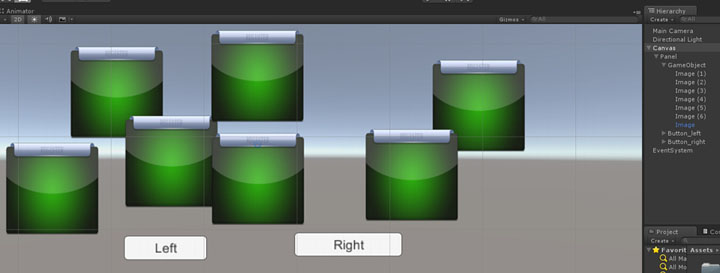•  添加UI按椭圆顺序排布脚本
• 新建脚本：Ellipse.cs脚本，对象绑定到Canvas对象。
• 编写代码:
//保存需要排序的精灵容器
public GameObject[] Sprites;
public Transform centerPoint;//椭圆的中心点
public float anglecheap = 25;//每个方块间的角度偏移
//保存位置点
private List<Vector3> location = new List<Vector3>();
private float angle =270;//第一个保证是中心位置的,当前到的角度
private float firstangle = 270;//记录第一个角度，用以左右对称
private float r =90;//椭圆的两个弦长
private float R = 150;
int size = 0;//即对象数组的一半，奇数为总长度+1/2,偶数为一半<pre name="code" class="csharp">// Use this for initialization
void Start ()
{//初始化size
if (Sprites.Length % 2 == 0)
{
size = Sprites.Length / 2;
}
else
{
size = (Sprites.Length + 1) / 2;
}
//排序分级显示
makespriteSort();
//重置渲染层级
ResetDeep();
}
//给这些精灵排序显示
void makespriteSort()
{
//取出椭圆的中心点
Vector3 center = centerPoint.position;
//判断该数组的个数奇偶性，如果是偶数，那么需要留出一个来放到对面
if (Sprites.Length % 2 == 0)
{
//右半边
for (int i = 0; i < size; i++)
{
Sprites[i].transform.position = getPosition(angle, center);
//       m_rightsprite.Add(Sprites[i]);
angle += anglecheap;
}
//第一个已经得是左边了
angle = firstangle - anglecheap;
//左半边
for (int i = size; i < Sprites.Length-1; i++)
{
Sprites[i].transform.position = getPosition(angle, center);
angle -= anglecheap;
//  m_leftsprite.Add(Sprites[i]);
}
//最后一个
Sprites[Sprites.Length - 1].transform.position = getPosition(firstangle-180, center);
// m_leftsprite.Add(Sprites[Sprites.Length - 1]);
return;
}
//如果不是偶数，那么出去中间那个，正好正常显示
else
{
//右半边
for (int i = 0;i<size; i++)
{
Sprites[i].transform.position = getPosition(angle, center);
//    m_rightsprite.Add(Sprites[i]);
angle += anglecheap;
}
//第一个已经得是左边了
angle = firstangle - anglecheap;
//左半边
for (int i = size;i < Sprites.Length; i++)
{
Sprites[i].transform.position = getPosition(angle, center);
//  m_leftsprite.Add(Sprites[i]);
angle -= anglecheap;
}
return;
}

}
//获取当前角度的坐标
Vector3 getPosition(float _angle,Vector3 _centerposition)
{
float hudu = (_angle/180f) * Mathf.PI;
float cosx = Mathf.Cos(hudu);
float sinx = Mathf.Sin(hudu);
float x = _centerposition.x + R * cosx;
float y = _centerposition.y + r * sinx;
Vector3 point = new Vector3(x, y, 0);
//添加到容器保存
location.Add(point);
return  point;
}

//根据当前左右容器调整所有控件的渲染层级
void ResetDeep()
{
dep = 0;
//右半边
for (int i =size-1; i>=0; i--)
{
Sprites[i].GetComponent<Transform>().SetSiblingIndex(dep);
dep++;
}
dep = 0;
//左半边
for (int i = Sprites.Length - 1; i >=size; i--)
{
Sprites[i].GetComponent<Transform>().SetSiblingIndex(dep);
dep++;
}
}
运行后可以看到如图效果（不要忘了把这几个对象拖到脚本里，中心点是传入的对象，所以有一个位置要摆好，摆在想要的中心点中，也可以自己改成手动输入x，y，z）：
•